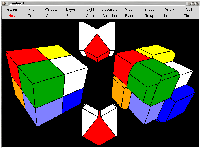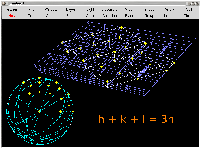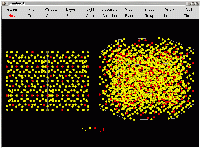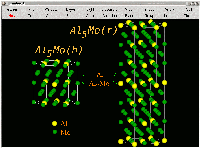GAMGI 0.11Normal and weighted Voronoi tesselation of a water molecule and a cube of different atoms (H, O, N, C, P, Si, Na... in increasing order of covalent radius, with colors white, red, light blue, green, orange, yellow, blue... respectively), always with periodic boundary conditions. Larger atoms have larger polyhedra in weighted (radical) Voronoi tesselation, changing completely the topology of the system. Normal and weighted tesselation systems are shown at the same scale, with a perspective projection. Size: 23,985 bytes.Reciprocal lattice and stereographic projection of a rhombohedral (hR) lattice (a = 1.0 c = 3.0), showing the planes (the yellow crosses) and directions (the green traces). The reciprocal lattice of the corresponding primitive (hP) lattice is also shown (in blue). In a hR lattice, planes with conventional indices must obbey the rule -h+k+l = 3n, which explains the absence of the other planes. The pole close to the center of the stereographic projection is the plane (003), which is above the origin (the node without plane) in the reciprocal lattice. Size: 37,089 bytes.Atomic structure of Al4Cr intermetallic compound, belonging to the hexagonal P6_3/mmc (194) space group, in perspective (right) and along direction  (left). Each cell (a = 1.998 nm, c = 2.467 nm, beta = 119.3 degrees) shows 1800 atoms, corresponding to 563 atoms inside the hexagonal prism, 453 of Al and 110 of Cr. Atoms partially in yellow and red correspond to positions statistically occupied by atoms of both elements, so the sum of the two occupancy numbers is 1.0. From Amélia Almeida, PhD thesis. Size: 80,443 bytes.Atomic structure of high- (above 800 C) and low-temperature (below 648 C) allotropic phases of Al5Mo intermetallic compound, Al5Mo(h) and Al5Mo(r), respectively. Al5Mo(h) has a hP lattice (a = 0.4912 nm, c = 0.8860 nm, space group = P6_3 = 173) while Al5Mo(r) is rombohedral (a = 0.4951 nm, c = 2.6231 nm, space group = R-3c = 167). From Amélia Almeida, PhD thesis. Size: 65,670 bytes.Crystallographic planes and directions in: 1) an orthorhombic body-centered lattice (a = 3.1 Angstrom, b = 3.0 Angstrom, c = 1.0 Angstrom), represented by a spherical volume (r = 5.0 Angstrom) and by 5 x 5 x 5 Wigner-Seitz cells; 2) the three cubic lattices (c = 1.0 Angstrom); 3) the hexagonal lattice (a = 1.0 Angstrom, c = 1.633 Angstrom). Size: 34,537 bytes.2 x 10 x 2 conventional cells (a = 748 pm, b = 255 pm, c = 497 pm) for orthorhombic high-density polyethylene (space group = 62, Wyckoff positions: 1) C c 0.0330, 0.0660; 2) H c 0.1898, 0.0520; 3) H c 0.0339, 0.2607). Chains (CH2)n are aligned along direction b (instead of direction c, as usual), to use the standard space group Pnma (instead of Pnam), as defined in the International Tables for Crystallography. Crystalline polyethylene can also be obtained experimentally with monoclinic symmetry (space group 12 = C2/m). Size: 25,763 bytes.

GAMGI old

Screenshots for older releases can be found here.